随机掺杂对超深亚微米SOI MOSFETs阈值电压影响研究Study on the Influence of Random Doping on the Threshold Voltage of Ultra Deep Submicron SOI MOSFETs

• 全文下载: PDF(2036KB)    PP.472-479   DOI: 10.12677/APP.2018.811060
• 下载量: 182  浏览量: 620   科研立项经费支持

In this paper, the threshold voltage fluctuations of ultra-deep submicron SOI MOSFETs caused by random dopant fluctuation are studied. An analytical model of the standard deviation of threshold voltage fluctuation based on the number fluctuation of channel particles is proposed, which takes into account both the variation of the number and position of channel particles. By studying the threshold voltage variations under different parameters, the predicted results calculated by the model are in good agreement with the numerical simulation results of Sentaurus TCAD.

1. 引言

2. 短沟道SOI MOSFETs阈值电压模型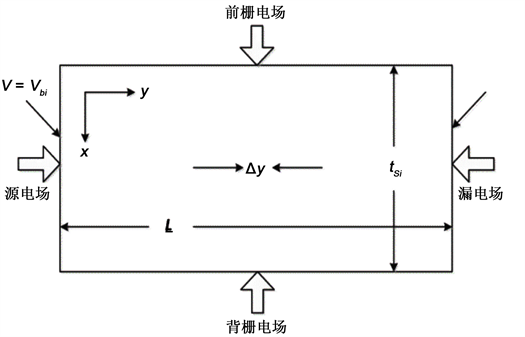Figure 1. Gaussian box method for solving threshold voltage

$\frac{{\partial }^{2}{\varphi }_{1}\left(x,y\right)}{\partial {x}^{2}}+\frac{{\partial }^{2}{\varphi }_{1}\left(x,y\right)}{\partial {y}^{2}}=\frac{q{N}_{A}}{{\epsilon }_{Si}}\text{\hspace{0.17em}}\text{\hspace{0.17em}}\left(0\le x\le {t}_{Si},0\le y\le L\right)$ (1)

$\frac{{\epsilon }_{Si}{t}_{Si}}{{\eta }_{1}}\frac{\partial {E}_{sf}\left(y\right)}{\partial y}+{C}_{ox}\left[{V}_{gs}-{V}_{fbf}-{\phi }_{sf}\left(y\right)\right]+{C}_{box}\left[{V}_{su}-{V}_{subbf}-{\phi }_{sb}\left(y\right)\right]=q{N}_{A}{t}_{Si}$ (2)

η1可以看作工艺常数，通过拟合得到。通过拟合参数η1来说明横向电场Esf(y)/η1的影响。tsi为硅膜区厚度，Vfbf、Vfbb分别为沟道正、背表面的平带电压。Cox、Csi、Cbox分别代表栅氧、硅膜和埋氧等效电容。

${V}_{th}={V}_{th,L}-\Delta {V}_{th,SCE}$ (3)

${V}_{th,L}={V}_{fbf}+\frac{{\stackrel{˜}{C}}_{box}}{{\stackrel{˜}{C}}_{ox}}{\phi }_{s}-\frac{{\stackrel{˜}{C}}_{box}}{{C}_{fox}}\left({V}_{sub}-{V}_{fbb}\right)+\left(1-\frac{{\stackrel{˜}{C}}_{box}}{2{C}_{si}}\right)\frac{q{N}_{A}{t}_{si}}{{C}_{fox}}$ (4)

$\text{Δ}{V}_{th,SCE}=\frac{{\stackrel{˜}{C}}_{box}}{{\stackrel{˜}{C}}_{ox}}×\frac{\left[2\left({V}_{bi}-2{\phi }_{f}\right)+{V}_{ds}\right]}{2\text{cosh}\left({L}_{g}/2l\right)-2}$ (5)

$\frac{1}{{\stackrel{˜}{C}}_{box}}=\frac{1}{{C}_{box}}+\frac{1}{{C}_{si}}$ (6)

$\frac{1}{{\stackrel{˜}{C}}_{fox}}=\frac{1}{{C}_{fox}}+\frac{1}{{C}_{si}}$ (7)

$\frac{1}{{\stackrel{˜}{C}}_{ox}}=\frac{1}{{C}_{fox}}+\frac{1}{{C}_{si}}+\frac{1}{{C}_{box}}$ (8)

3. 反应沟道粒子数目波动和位置变化的阈值电压标准差模型

${\left(\sigma {V}_{th,total}\right)}^{2}={\left(\sigma {V}_{th,pos}\right)}^{2}+{\left(\sigma {V}_{th,num}\right)}^{2}$ (9)

3.1. 粒子数目波动引起的阈值电压波动

$\begin{array}{c}{V}_{th,L}={{\varphi }^{\prime }}_{m,f}-\left[{{\chi }^{\prime }}_{f}+\frac{{E}_{g}}{2q}+\frac{kT}{q}\mathrm{ln}\left(\frac{{N}_{A}}{{n}_{i}}\right)\right]-\frac{{Q}_{ss,f}}{{C}_{fox}}+2\frac{{\stackrel{˜}{C}}_{box}}{{\stackrel{˜}{C}}_{ox}}\frac{kT}{q}\mathrm{ln}\left(\frac{{N}_{A}}{{n}_{i}}\right)\\ \text{\hspace{0.17em}}\text{\hspace{0.17em}}-\frac{{\stackrel{˜}{C}}_{box}}{{C}_{fox}}\left({V}_{sub}+\frac{kT}{q}\mathrm{ln}\frac{{N}_{sub}}{{N}_{A}}\right)+\left[1+\frac{{\stackrel{˜}{C}}_{box}}{{C}_{si}}\right]\frac{q{N}_{A}{t}_{si}}{{C}_{fox}}\end{array}$ (10)

${V}_{th,L}={A}_{1}\left[\mathrm{ln}\frac{{\stackrel{¯}{N}}_{A}}{{n}_{i}}+\frac{1}{{\stackrel{¯}{N}}_{A}}\left({N}_{A}-{\stackrel{¯}{N}}_{A}\right)\right]+{A}_{2}{N}_{A}+{A}_{3}\left[\mathrm{ln}\frac{{\stackrel{¯}{N}}_{A}}{{N}_{sub}}+\frac{1}{{\stackrel{¯}{N}}_{A}}\left({N}_{A}-{\stackrel{¯}{N}}_{A}\right)\right]+{B}_{1}$ (11)

${A}_{1}=\frac{kT}{q}\left(\frac{2{\stackrel{˜}{C}}_{box}}{{\stackrel{˜}{C}}_{ox}}-1\right)$ (12)

${A}_{2}=\frac{q{t}_{si}}{{C}_{fox}}\left[1-\frac{{\stackrel{˜}{C}}_{box}}{2{C}_{si}}\right]$ (13)

${A}_{3}=\frac{kT}{q}\frac{{\stackrel{˜}{C}}_{box}}{{C}_{fox}}$ (14)

${B}_{1}={{\varphi }^{\prime }}_{m,f}-{{\chi }^{\prime }}_{f}-\frac{{E}_{g}}{2q}-\frac{{Q}_{ss,f}}{{C}_{fox}}-\frac{{\stackrel{˜}{C}}_{box}}{{\stackrel{˜}{C}}_{fox}}\left({V}_{sub}-{V}_{fbb}\right)$ (15)

$\begin{array}{c}{V}_{th}=\left(\frac{{A}_{1}+{A}_{3}}{{\stackrel{¯}{N}}_{A}}+{A}_{2}+\frac{{A}_{4}}{{\stackrel{¯}{N}}_{A}}\right){N}_{A}+{A}_{1}\left(\mathrm{ln}\frac{{\stackrel{¯}{N}}_{A}}{{n}_{i}}-1\right)+{A}_{3}\left(\mathrm{ln}\frac{{\stackrel{¯}{N}}_{A}}{{N}_{sub}}-1\right)\\ \text{\hspace{0.17em}}\text{\hspace{0.17em}}-{A}_{4}\left(\mathrm{ln}\frac{{N}_{D}{\stackrel{¯}{N}}_{A}}{{n}_{i}^{2}}+1-2\mathrm{ln}\frac{{\stackrel{¯}{N}}_{A}}{{n}_{i}}\right)+{B}_{1}-{B}_{2}\end{array}$ (16)

${A}_{4}=\frac{kT}{q}\frac{{\stackrel{˜}{C}}_{box}}{{\stackrel{˜}{C}}_{ox}\left(\text{cosh}\left({L}_{g}/2l\right)-1\right)}$ (17)

${B}_{2}=\frac{{V}_{ds}{\stackrel{˜}{C}}_{box}}{2{\stackrel{˜}{C}}_{ox}\left(\text{cosh}\left({L}_{g}/2l\right)-1\right)}$ (18)

$\sigma {V}_{th,num}=\left(\frac{{A}_{1}+{A}_{3}}{{\stackrel{¯}{N}}_{A}}+{A}_{2}+\frac{{A}_{4}}{{\stackrel{¯}{N}}_{A}}\right)\sqrt{\frac{{\stackrel{¯}{N}}_{A}}{v}}=\left(\frac{{A}_{1}+{A}_{3}+{A}_{4}}{{\stackrel{¯}{N}}_{A}}+{A}_{2}\right)\sqrt{\frac{{\stackrel{¯}{N}}_{A}}{WL{t}_{si}}}$ (19)

3.2. 粒子位置波动引起的阈值电压波动

${R}_{p}={\left(\frac{\sigma {V}_{th,pos}}{\sigma {V}_{th}}\right)}^{2}$ (20)

${\left(\sigma {V}_{th,total}\right)}^{2}={\left(\sigma {V}_{th,pos}\right)}^{2}+{\left(\sigma {V}_{th,num}\right)}^{2}$ (21)

${R}_{p}={R}_{p0}+{C}_{0}\cdot {\mathrm{log}}_{10}{\left({L}_{g}{N}_{A}^{1/2}\right)}^{-1}$ (22)

4. 结果与分析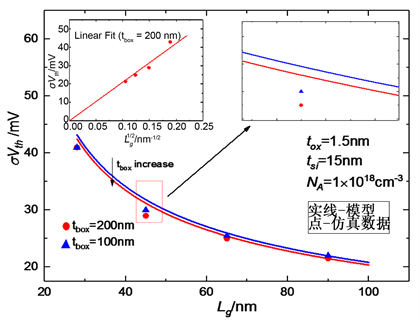Figure 2. σVth versus channel length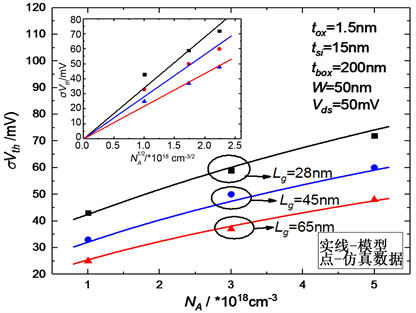Figure 3. σVth versus channel length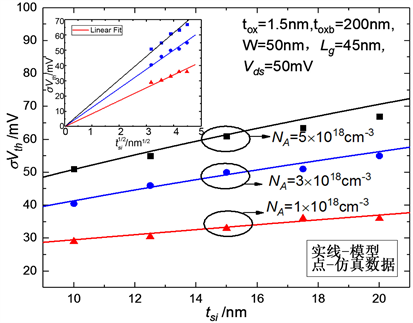Figure 4. σVth versus silicon film thickness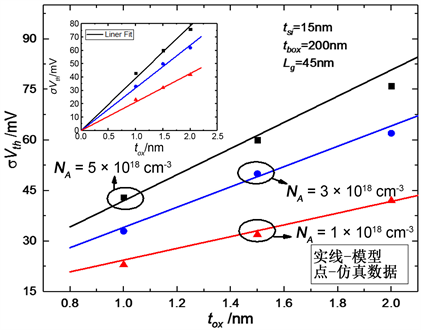Figure 5. σVth versus gate oxide thickness

5. 结论

  孔文. 全耗尽绝缘硅FD-SOI工艺技术的特点分析[J]. 集成电路应用, 2017, 34(11): 38-41.  Sun, X., Moroz, V., Damrongplasit, N., et al. (2011) Variation Study of the Planar Ground-Plane Bulk MOSFET, SOI FinFET, and Trigate Bulk MOSFET Designs. IEEE Transactions on Electron Devices, 58, 3294-3299. https://doi.org/10.1109/TED.2011.2161479  Mazurier, J., Weber, O., Andrieu, F., et al. (2011) On the Varia-bility in Planar FDSOI Technology: From MOSFETs to SRAM Cells. IEEE Transactions on Electron Devices, 58, 2326-2336. https://doi.org/10.1109/TED.2011.2157162  Markov, S., Cheng, B. and Asenov, A. (2012) Sta-tistical Variability in Fully Depleted SOI MOSFETs Due to Random Dopant Fluctuations in the Source and Drain Ex-tensions.  IEEE Electron Device Letters, 33, 315-317. https://doi.org/10.1109/LED.2011.2179114  Chatzikyriakou, E., White, W.R. and Groot, C.H.D. (2017) Total Ionizing Dose, Random Dopant Fluctuations, and Its Combined Effect in the 45 nm PDSOI Node. Microelectronics Reliability, 68, 21-29. https://doi.org/10.1016/j.microrel.2016.11.007  Lü, W., Wang, G. and Sun, L. (2015) Analytical Modeling of Cutoff Frequency Variability Reserving Correlations Due to Random Dopant Fluctuation in Nanometer MOSFETs. Solid-State Electron, 105, 63-69. https://doi.org/10.1016/j.sse.2014.12.024  Andrei, P. and Oniciuc, L. (2008) Suppressing Random Do-pant-Induced Fluctuations of Threshold Voltages in Semiconductor Devices. Journal of Applied Physics, 104, 1-10. https://doi.org/10.1063/1.2973457  Yasuda, Y., Takamiya, M. and Hiramoto, T. (2000) Separation of Effects of Statistical Impurity Number Fluctuations and Position Distribution on Vth Fluctuations in Scaled MOSFETs. IEEE Transactions on Electron Devices, 47, 1838-1842. https://doi.org/10.1109/16.870557  Rao, R., DasGupta, N. and DasGupta, A. (2010) Study of Random Dopant Fluctuation Effects in FD-SOI MOSFET Using Analytical Thresh-old Voltage Model. IEEE Transactions on Device and Materials Reliability, 10, 247-253. https://doi.org/10.1109/TDMR.2010.2044180  Banna, S.R., Chan, P.C.H., Ko, P.K., et al. (1995) Threshold Voltage Model for Deep-Submicrometer Fully Depleted SOI MOSFET’s. IEEE Transactions on Electron Devices, 42, 1949-1955. https://doi.org/10.1109/16.469402